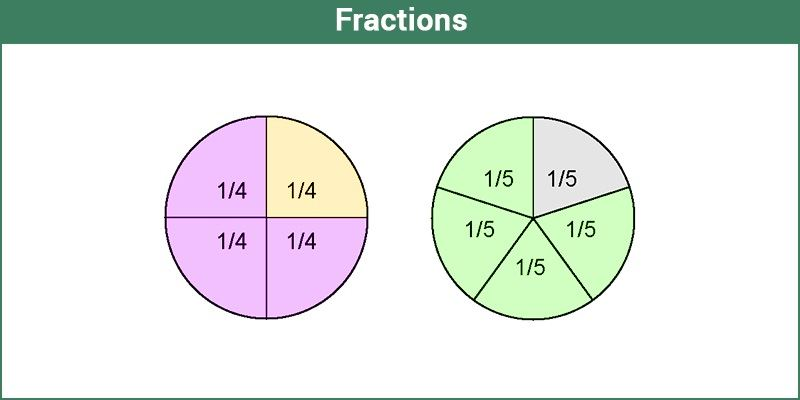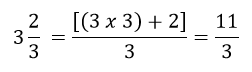# Fractions

In Mathematics, fractions are defined as the parts of a whole. The whole can be a number or any specific value or a thing. In other words, it is also termed as a portion or section of any quantity. It is denoted by using ‘/’ symbol, such as a/b. For example, in 2/4 is a fraction where the upper part denotes the numerator and lower part is the denominator.

A fraction is a word that is originated from Latin. In Latin, “Fractus” means “broken”. In real life, when we cut a piece of cake from the whole of it, say 1/4th of it, then the portion is the fraction of the cake.## Definition of Fractions

A fraction represents a numerical value, which defines the parts of a whole. Suppose a number has to be divided into four parts, then it is represented as x/4. So the fraction here, x/4, defines 1/4th of number x. Fractions form an important part of our daily lives. There are many examples of fractions you will come across in real life. We have to willingly or unwillingly share that yummy pizza amongst our friends and families. Three people, four slices.

If you learn and visualize fractions in an easy way, it will be more fun and exciting. Slice an apple, and we get fractions.

Also, Check:

## Type of Fractions

Based on the properties of numerator and denominator, fractions are sub-divided into different types. They are:

• Proper fractions
• Improper fractions
• Mixed fractions
• Like fractions
• Unlike fractions
• Equivalent fractions

### Proper Fractions

The proper fractions are those where the numerator is less than the denominator. For example, 8/9 will be a proper fraction since “numerator < denominator”.

### Improper Fractions

The improper fraction is a fraction where the numerator happens to be greater than the denominator. For example, 9/8 will be an improper fraction since “denominator < numerator”.

### Mixed Fractions

A mixed fraction is a combination of the integer part and a proper fraction. These are also called a mixed number or mixed numeral. For example:### Like Fractions

Like fractions are those fractions, as the name suggests, that are alike or same.

For example, take ½ and 2/4; they are alike since if you simplify it mathematically, you will get the same fraction.

### Unlike Fractions

Unlike fractions, are those that are dissimilar.

For example, ½ and 1/3 are unlike fractions.

### Equivalent Fractions

Two fractions are equivalent to each other if after simplification either of two fractions is equal to the other one.

For example, ⅔ and 4/6 are equivalent fractions.

Since, 4/6 = (2×2)/(2×3) = 2/3

## Unit Fractions

A fraction is known as a unit fraction, when the numerator is equal to 1.

• One half of whole = ½
• One-third of whole = 1/3
• One-fourth of whole = ¼
• One-fifth of whole = ⅕

## Properties

Similar to real numbers and whole numbers, a fractional number also holds some of the important properties. They are:

• Commutative and associative properties hold true for fractional addition and multiplication
• The identity element of fractional addition is 0, and fractional multiplication is 1
• The multiplicative inverse of a/b is b/a, where a and b should be non zero elements
• Fractional numbers obey the distributive property of multiplication over addition

## Fractions Rules

There are some rules we should know before solving the problems based on fractions.

Rule #1: Before adding or subtracting of fractions, we should make sure that the denominators are equal. Hence, addition and subtraction of fractions are possible with a common denominator.

Rule #2: When we multiply two fractions, then the numerators are multiplied as well as the denominators are multiplied. Later simplify the fraction.

Rule #3: When we divide a fraction from another fraction, we have to find the reciprocal of another fraction and then multiply with the first one to get the answer.

Addition of fractions is easy when they have a common denominator.

For example, ⅔ + 8/3 = (2+8)/3 = 10/3

Hence, we need to just add the numerators here.

If the denominators of the two fractions are different, we have to simplify them by finding the LCM of denominators and then making it common for both fractions.

Example: ⅔ + ¾

The two denominators are 3 and 4

Hence, LCM of 3 and 4 = 12

Therefore, multiplying ⅔ by 4/4 and ¾ by 3/3, we get;

8/12 + 9/12

= (8+9)/12

= 17/12

## Subtracting Fractions

The rule for subtracting two or more fractions is same as for addition. The denominators should be common to subtract two fractions.

Example: 9/2 – 7/2 = (9-7)/2 = 2/2 = 1

### Subtracting with Different Denominators

If the denominators of the two fractions are different, we have to simplify them by finding the LCM of denominators and then making it common for both fractions.

Example: ⅔ – ¾

The two denominators are 3 and 4

Hence, LCM of 3 and 4 = 12

Therefore, multiplying ⅔ by 4/4 and ¾ by 3/3, we get;

8/12 – 9/12

= (8-9)/12

= -1/12

## Multiplication of Fractions

As per rule number 2, we have discussed in the previous section, when two fractions are multiplied, then the top part (numerators) and the bottom part (denominators) are multiplied together.

If a/b and c/d are two different fractions, then the multiplication of a/b and c/d will be:

(a/b) x (c/d) = (axc)/(bxd) = (ac/bd)

Example: Multiply ⅔ and 3/7.

(⅔) x (3/7) = (2×3)/(3×7) = 2/7

## Division of Fractions

If we have to divide any two fractions, then we will use here rule 3 from the above section, where we need to multiply the first fraction to the reciprocal of the second fraction.

If a/b and c/d are two different fractions, then the division a/b by c/d can be expressed as:

Example: Divide ⅔ by 3/7.

(⅔) ÷ (3/7) = (⅔) x (7/3) = (2×7)/(3×3) = 14/9

### Real-Life Examples of Fractions

Let us visualize some of the fractions examples:

1. Imagine a pie with four slices. Taking two slices of pie for yourself would mean that you have two out of the four. Hence, you represent it as 2/4.
2. Fill half a glass of water. What do you see? 1/2 glass is full. Or 1/2 glass is empty. This 1/2 is fractions where 1 is the numerator that is, the number of parts we have. And 2 is the denominator, the number of parts the whole glass is divided into.## Fractions To Decimals

As we already learned enough about fractions, which are the part of a whole. The decimals are the numbers expressed in a decimal form which represents fractions, after division.

For example, Fraction 1/2 can be written in decimal form as 0.5.

The best part of decimals are they can be easily used for any arithmetic operations such as addition, subtraction, etc. Whereas it is difficult sometimes to perform operations on fractions. Let us take an example to understand;

solution: 1/6 = 0.17 and 1/4 = 0.25

Hence, on adding 0.17 and 0.25, we get;

0.17 + 0.25 = 0.42

## Solved Examples

Question 1: Is 12/6 a fraction?

Solution: Yes, it is. It is called an improper fraction.

Question 2: Convert 130.1200 into a fraction.

Solution: Here will use the concept of how to convert decimals into fractions

130.1200 = 130.1200/10000

= 13012/100

Question 3: Add 3/ 5 and 10/15.

Solution:

3 /5 + 10/15

LCM of 5 and 15 is 15

= (9 + 10)/15

= 19/15

### Video Lesson## Fractions Worksheets

Solve the following:

1. 3/7+9/2-8
2. 22/7+8/11
3. 32/9 x 81/4
4. 44/9 ÷ 36/4

## Frequently Asked Questions – FAQs

### What are fractions?

Fractions are the numerical values that are a part of whole. If a number or a thing is divided into equal parts, then each part will be the fraction of whole. A fraction is denoted as a/b, where a is the numerator and b is the denominator.

### How to solve fractions?

To add or subtract fractions, we have to check if the denominators are same or different. For same denominators, we can directly add or subtract the numerators, keeping the denominator common. But if the denominators are different, then we need to simplify them by finding the LCM.

### What are the 3 types of fractions in Maths?

The 3 types of fractions in Maths are: Proper fractions, Improper fractions and Mixed fractions.

### Give real life examples of fractions.

If a watermelon is divided into four equal parts, then each part is a fraction of ¼.
Similarly, if a pizza is divided into three equal parts, then each part shows 1/3rd of pizza.

### What is a unit fraction?

A fraction with numerator 1 is called unit fraction. Examples are: ½, ⅓, ¼, ⅕, 1/7, 1/10, etc.

Quiz on Fractions

1. Punyavathi

Nice explanation

2. anuvansh

nice explanayion mam that makeme understand the topic

3. Ayush Das

Thanks, nice video 👍I learner to the end teachers. It’s interesting I liked it . Thanks for sending .

Hats off to you

5. Shalini Kale

Oh it’s so helpful thanks again

6. If 1/2 + 1/x = 2, then x =what?

1. lavanya

Given,
1/2 + 1/x = 2
Rationalising the denominator, we get;
(x+2)/2x = 2
x+2 = 4x
3x = 2
x = 2/3